# Shape theory

(diff) ← Older revision | Latest revision (diff) | Newer revision → (diff)

The fundamental notions of homotopy theory can be successfully applied only to spaces whose local behaviour is sufficiently regular, e.g. to manifolds, polyhedra, CW-complexes, and ANR spaces. Shape theory is a modification of homotopy theory designed to give satisfactory results for arbitrary topological spaces, especially for metric compacta. When restricted to spaces with regular local behaviour, shape theory coincides with homotopy theory; therefore it can be viewed as the appropriate extension of homotopy theory to general spaces.

Many constructions in topology lead naturally to spaces with bad local behaviour even when one initially considers manifolds. Standard examples include fibres of mappings, sets of fixed points, remainders of compactifications, attractors of dynamical systems, spectra of operators, or boundaries of certain groups. In all these areas, shape theory has proved useful. In particular, it has applications in the study of cell-like mappings, approximate fibrations and shape fibrations, imbeddings of compacta in Euclidean spaces and in exact homology theories.

Shape theory became a separate area of topology when K. Borsuk defined the shape category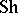of compact metric spaces and the shape functor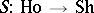defined on the homotopy category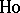(of metric compacta) [a2]. The shape category was soon extended to arbitrary topological spaces [a17]. The standard approach to the construction ofconsists in replacing spaces by admissible inverse systems of polyhedra or ANR spaces, called expansions, and in developing a homotopy theory of such systems. In the case of compact Hausdorff spaces(cf. also Compact space), as an expansion one can use any inverse system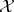whose limit is, [a14]. In the shape category, objects are topological spaces (cf. Topological space), while the morphisms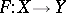are given by morphisms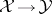between polyhedral expansions ofand, respectively, which belong to the category pro-, associated with the homotopy category [a8].

One of the first successful applications of shape theory is Fox's theory of overlays, a modification of covering space theory [a7]. While the classical classification theorem of covering spaces requires local connectedness and semi-local-connectedness of the base space, the corresponding result in shape theory is valid for all metric spaces with covering spaces replaced by overlays [a7]. The role of the fundamental group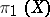is taken up by the fundamental pro-group of. This is the inverse system of groups obtained from a polyhedral expansion ofupon application of the functor. Other classical theorems of homotopy theory also have their shape-theoretic versions. In particular, this includes the theorems of J.H.C. Whitehead, W. Hurewicz and S. Smale. The statements of these results also use pro-groups, in particular the homology and the homotopy pro-groups. In the case of the Whitehead theorem, a mappingbetween pointed finite-dimensional topological spaces is a shape equivalence, i.e., an isomorphism of pointed shape, if and only if it induces isomorphisms of all homotopy pro-groups. In contrast to the classical theorem, which refers to CW-complexes (cf. also CW-complex), there are no such restrictions in the shape-theoretic version [a16], [a12], [a4].

In 1972, T.A. Chapman considered compactawhich are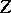-imbedded in the Hilbert cube, i.e., have the property that there exist mappings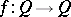that are arbitrarily close to the identity while their image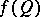misses. Chapman's complement theorem asserts that two compacta,, imbedded inas-sets, have the same shape if and only if their complements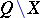,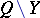are homeomorphic. There also exist finite-dimensional complement theorems, where the ambient space is the Euclidean space. Compactaandare required to be "nicely" imbedded, i.e., satisfy the inessential loops condition and satisfy appropriate dimensional and shape-connectedness conditions [a10].

Chapman's work also led to the discovery of the strong shape categoryand the strong shape functor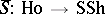. D.A. Edwards and H.M. Hastings established an isomorphism between the category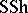of-sets of the Hilbert cube and the proper homotopy category of their complements [a6]. Strong shape theory has a richer structure than the usual shape theory, i.e., there exists a forgetful functor(cf. also Functor) which relates the two theories. The strong shape category occupies an intermediate place between the homotopy category and the usual shape theory, i.e., the shape functoradmits a factorization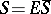.

The construction ofand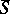for arbitrary topological spaces requires a careful choice of admissible expansions, the so-called strong expansions [a13]. Moreover, the correct homotopy theory of inverse systems is coherent homotopy theory [a1], [a11].

The following result is a concrete example of successful applications of shape theory. A finite-dimensional compactum imbeds in a (differentiable) manifoldas an attractor of a (smooth) flow (continuous-time dynamical system) onif and only if it has the shape of a compact polyhedron [a9].

How to Cite This Entry:
Shape theory. Encyclopedia of Mathematics. URL: http://encyclopediaofmath.org/index.php?title=Shape_theory&oldid=18369
This article was adapted from an original article by S. MardeÅ¡iÄ‡ (originator), which appeared in Encyclopedia of Mathematics - ISBN 1402006098. See original article